# An Introduction to Polar Coordinates

##### Age 14 to 18

Published 2005 Revised 2019

In one sense it might seem odd that the first way we are taught to represent the position of objects in mathematics is using Cartesian coordinates when this method of location is not the most natural or the most convenient. For a start, you have to use negative as well as positive numbers to describe all the points on the plane and you have to create a grid (well axes) to use as a reference.

When you ask a child where they left their ball they will say "just over there'' and point. They are describing (albeit very roughly) a distance "just'' and a direction "over there'' (supported by a point or a nod of the head). When you ask someone where their town is they often say things like "about $30$ miles north of London''. Again, a distance and direction. It is not very often that someone gives the latitude and longitude of their town!

The use of a distance and direction as a means of describing position is therefore far more natural than using two distances on a grid. This means of location is used in polar coordinates and bearings.

The polar coordinates of a point describe its position in terms of a distance from a fixed point (the origin) and an angle measured from a fixed direction which, interestingly, is not "north'' (or up on a page) but "east'' (to the right). That is in the direction $Ox$ on Cartesian axes.

So:

In the plane we choose a fixed point $O$, known as "the pole''.

Then we choose an axis $Ox$ through the pole and call it the "polar axis".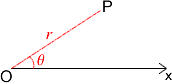We now need a way of describing these points in a way that is efficient and understood by everyone.
We say that $(r,\theta )$ are the polar coordinates of the point $P$, where $r$ is the distance $P$ is from the origin $O$ and $\theta$ the angle between $O x$ and $O P$.

Here are some points on a plane and a list of five sets of Polar coordinates. Can you match the points to their coordinates (answers at the end of the article). I have added some circles to help with the distances.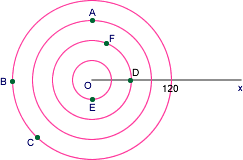$$\begin{eqnarray} (60,0)\\ (30, 270)\\ (120, 225)\\ (90, 90)\\ (60,60)\\ (120, 180) \end{eqnarray}$$

So far I have measured the angles in degrees $(^\circ)$ but the normal convention is to use radians $(^c)$. There are $2\pi$ radians in a full turn. That is:

$$\begin{eqnarray} 360^\circ = 2\pi^c\\ 180^\circ =\pi^c\\ 90^\circ = \left(\frac{\pi}{2}\right) ^c\\ 1^\circ = \left( \frac{2\pi}{360}\right) ^c \end{eqnarray}$$

Looking at one of our points in the list above $(90,90)$ would be $(90,\frac{\pi}{2})$ if the angle is measured in radians.

Can you write each of the other pairs of polar coordinates above using radian measure? (Answers at the end of the article).

There are in fact an infinite number of ways you can write any point using polar coordinates because you can always add $2\pi^c$, or $4\pi^c$, or $6\pi^c$ ... to the angle and still end up pointing in the same direction! In the example above the general coordinates for $A$ would be $(90,2n\pi + \frac{\pi}{2})$, where $n$ is an integer.

This also means that the polar coordinates of the pole $O$ are $(0,\theta)$ where $\theta$ can be any angle.

### The Relationship Between Polar and Cartesian Coordinates

Imagine a point $P$ which has polar coordinates $(r,\theta)$. Let's try to use this information to give the Cartesian coordinates of $P$ We can drop a perpendicular from the point $P$ to $Ox$ meeting $Ox$ at $Q$. The lengths of $OQ$ and $OP$ represent the $x$ and $y$ coordinates, in Cartesian form, so we just need to find these two distances.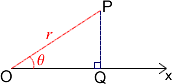$$\begin{eqnarray} PQ &=& r \sin \theta \\ OQ &=& r \cos \theta \end{eqnarray}$$

Therefore the Cartesian coordinates of $P$ are $(r \sin \theta, r \cos \theta)$

Now let's work the other way:

We will take the Cartesian coordinates of $P$ as $(x,y)$.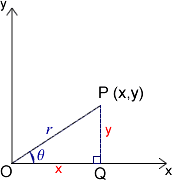Now we are trying to find $r$ and $\theta$ in terms of $x$ and $y$.

$$r = \sqrt{(x^2 + y^2)}$$

Now we need $\theta$ such that $x = r \cos \theta$ and $y = r \sin \theta$.

It is tempting to say that $\tan\theta = \frac{y}{x}$ but there is a danger in doing this that you might end up with the wrong value of $\theta$ because of the signs of the trigonometric ratios in each quadrant.

For example:

If $P$ were the point $(-2,-2)$ you would obtain a value for $\tan \theta = 1$ and you would not know whether $\theta = 45^\circ (\frac{\pi}{4}^c)$ or $225^\circ (\frac{3\pi}{2}^c)$!!

By using the signs of $\sin\theta$ and $\cos\theta$, you can be sure you have the angle in the correct quadrant.

So let's end by using this coordinate system. It would be good to try out some equations and look at their graphs (polar plots).

Let's look at some examples:

Consider the graph:

$r= \theta$

This has a spiral shape (each point moves out from the centre as the angle grows).

The diagram below shows graphs of $r= a\theta$ for different values of $a$, can you work out what they are?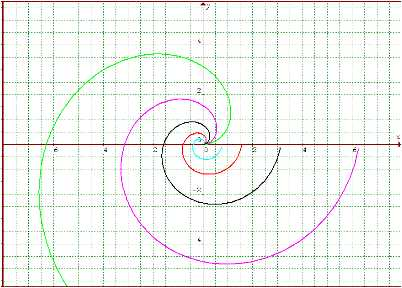Now its your turn. A graphical calculator or graphing package would be very helpful!

What would the series of graphs

$r=1, r=2, r=3,$... look like?

How about $r = 2a(1 + \cos\theta )$ for different values of $a$? These graphs are called cardioids by the way.

$$\begin{eqnarray} \mbox{D }\rightarrow(60,0)\\ \mbox{E }\rightarrow(30, 270)\\ \mbox{C }\rightarrow(120, 225)\\ \mbox{A }\rightarrow(90, 90)\\ \mbox{F }\rightarrow(60,60)\\ \mbox{B }\rightarrow(120, 180) \end{eqnarray}$$ And $$\begin{eqnarray} (60,0)\rightarrow(60,0)\\ (30, 270)\rightarrow(30, \frac{3\pi}{2})\\ (120, 225)\rightarrow(120, \frac{5\pi}{4})\\ (90, 90)\rightarrow(90, \frac{\pi}{2})\\ (60,60)\rightarrow(60,\frac{\pi}{3})\\ (120, 180)\rightarrow(120,\pi) \end{eqnarray}$$

### . . . . how to use an xy plot to help visualise the polar plot

When you are trying to imagine what the polar plot of a function will look like it can sometimes be helpful to look first at the Cartesian (xy) plot for that function using values $0$ to $2\pi$ ($0$ to $360$ degrees) and then imagine the graph turned into a fan.

Picture the x-axis pulled into a point with the function values fanned out around it.

For example : $y = 5 \sin 2x$ looks like this as a Cartesian plot.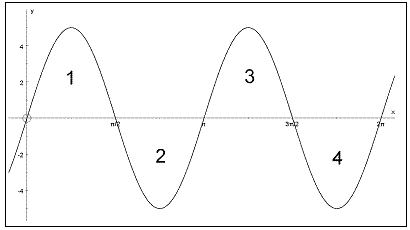But as a polar plot $r = 5\sin 2\theta$ is: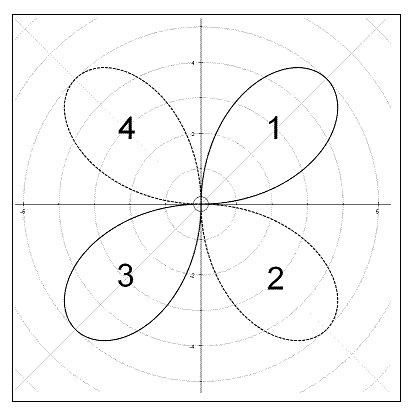Over the $0$ to $2\pi$ interval the graph $\sin 2x$ has $4$ regions
In region $1$ the function rises to a maximum value of $5$ and then falls back symmetrically to zero.

In region $2$ the function falls further to a minimum value of $-5$ before climbing back to zero.

Notice the position of region $2$ on the polar plot: as $\theta$ sweeps the second quadrant $\pi/2$ to $\pi$ the values of r are all negative, projecting each plot point backwards into the fourth quadrant.

Region $3$ is straightforward like region $1$, while region $4$, like region $2$, also has negative $r$ values and therefore plots in the second quadrant.

Now try $r = 5 + 5 \sin 2\theta$ :

First sketch an xy plot, then scrunch it up to make a fan round a point. After you have sketched the polar plot use a graphical calculator or a graph plotter to confirm your drawing.

Invent some functions of your own to play with. For example suppose I stay with the form $r = A + 5 \sin 2\theta$ , $A$ was $0$ initially then $5$. How will the appearance of the polar plot be effected by varying values of $A$ ?

Have fun.

Jennifer Piggott and Graeme Brown